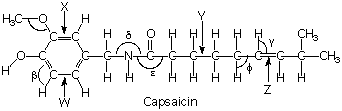Question# The structure of capsaicin, the molecule that is responsible for the "heat" in chili peppers, is shown below.

Other
ANSWEREDThe structure of capsaicin, the molecule that is responsible for the "heat" in chili peppers, is shown below.What are the approximate bond angles for the labeled angles? $$\displaystyle\beta=?,\delta,=?,\epsilon=?,\phi=?,\gamma=?$$2021-03-03
$$\displaystyle\beta={120}$$. There are 3 bonds and no unshared pairs, so itsgeometry is trigonal
$$\displaystyle\delta={104.5}$$ There are 3 bonds and one unshared pair,resulting in a "squished" tetrahedral geometry
$$\displaystyle\epsilon={120}$$ Another trigonal geometry
$$\displaystyle\phi={109.5}$$ This is a standard tetrahedral geometry,occurring when carbon bonds to 4 atoms
$$\displaystyle\gamma={120.3}$$ 3 bonded atoms with no unshared pairs
Bonds X and W are the same length because of resonance. Electrons are shared equally among the 6 carbons in the ring.
Bond X is longer than bond Z because because bond Z is a doublebond, while X is closer to a 1.5 bond. Higher bond orderspull the atoms closer together.
Bond Y is longer than bond Z for essentially the same reason as above.